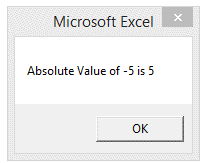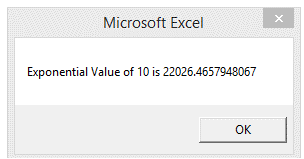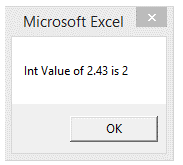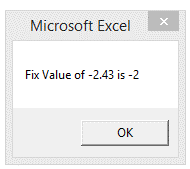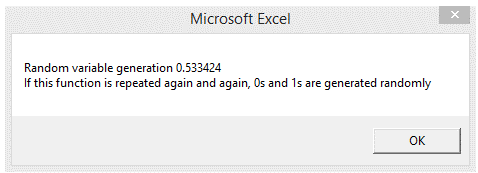## Vba math functions in Excel

Though its possible to perform basic arithmetic calculations using the arithmetic operators discussed before, there are some occasions,

when there is a need to perform more complicated mathematical operations.Here is where, The built-in Math functions of VBA comes to help.

The most common Math functions are as discussed below

1.Abs() function

This function returns the absolute value, that is, positive value of the given number.

2.Exp() funtion

This function returns the exponential of a given number.

3.Int() and Fix() function

These functions are used to truncate decimal part of the given number and return an integer.

The diffrence between Int() and Fix() is that, when the number is negative, the Fix() function

returns the smallest number larger than the integer.

4.Rnd()

This function is used to generate a random value which lies between 0 and 1.

This function is useful with projects dealing with Probability.

The following example illustrates the above functions

1. Sub math_func()
2. Dim i As Integer
3. Dim j As Double
4. i = -5
5. MsgBox "Absolute Value of " & i & " is " & (Abs(i))
6. i = 10
7. MsgBox "Exponential Value of " & i & " is " & (Exp(i))
8. j = 2.43
9. MsgBox "Int Value of " & j & " is " & (Int(j))
10. j = -2.43
11. MsgBox "Fix Value of " & j & " is " & (Fix(j))
12. MsgBox "Random variable generation " & Rnd() & vbNewLine & _
13. If this function is repeated again and again, 0s and 1s are generated randomly
14. End Sub

The screenshot: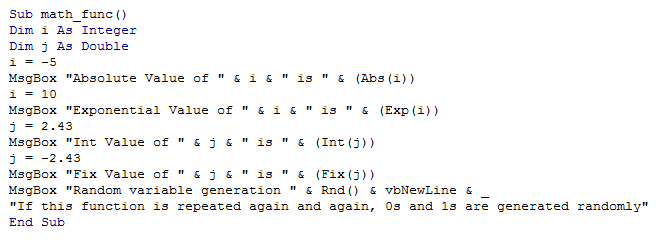Output: